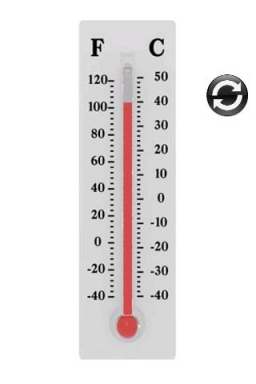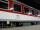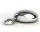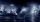# Temperature change 2

The outside temperature changed -14F over 4 hours. If the temperature changed the same amount each hour, what was the change in temperature each hour?

Correct result:

d =  3.5 °F

#### Solution:

$d=14\mathrm{/}4=\frac{7}{2}={\frac{7}{2}}^{\circ }\text{F}=3.{5}^{\circ }\text{F}$We would be pleased if you find an error in the word problem, spelling mistakes, or inaccuracies and send it to us. Thank you!## Next similar math problems:Added together and write as decimal number: LXVII + MLXIV
• The resultHow many times I decrease the number 1632 to get the result 24?
• DoctorsIn the city operates 196 doctors. The city has 134456 citizens. How many citizens are per one doctor?
• Math classificationIn 3A class are 27 students. One-third got a B in math and the rest got A. How many students received a B in math?
• Flood waterFlood waters in some US village meant that the homes had to evacuate 364 people. 50 of them stayed at elementary schools, 59 them slept with their friends and others went to relatives. How many people have gone to relatives?
• Cheaper carsState-owned railway company buys 10 air-conditioned coaches for nearly 18 million euros. Calculate how many euros is the equivalent of one seat in the wagon with a capacity of 83 people. How many of used cars at the price 2700 Euros can be buy instead of
• Celsius 25 degrees Celsius at midday dropped 12°Celsius degree by evening. What is the temperature?
• Melting pointThe melting point of mercury is -36°F and its boiling point is 672°F. What is the difference between the boiling point and the melting point?
• The temperature 6The temperature was 47°F on Thursday and 60°F on Friday. How much did the temperature rise?
• Storm 3If the temperature yesterday was 56 and today is 13 degrees cooler, what is today's temperature?
• The temperature 8The temperature on Monday was –6°C. On Tuesday the temperature was 3 degrees lower. Write down the temperature on Tuesday.
• The temperature 2The temperature of Siberia fell13 degrees below 2/3 degrees centigrad. What is the temperature there now?
• Change in temperatureStarting temperature is 21°C, the highest temperature is 32°C. What is the change in temperature?
• Difference of temperaturesThe temperature on Saturday was –2°C. The temperature on Sunday was 8°C. Write down the difference in these two temperatures.
• Temperature change 3At 2 p. M. The temperature was 76 degrees Fahrenheit. At 8 p. M. The temperature was 58 degrees Fahrenheit. What was the change in temperature?In about 12 hours in North Dakota the temperature rose from -33 degrees farenheit to 50 degrees farenheit. By how much did the temperature change?Today's temperature was 80 degrees, and then the temperature dropped 10 degrees. Then it dropped 15 degrees again, then the next day, the temperature went up 2 degrees. What would the temperature be?